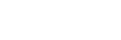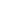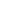# algorithm-base | 专门为刚开始刷题的同学准备的算法基地，没有最细只有更细，立志用动画将晦涩难懂的算法说的通俗易懂！ | Learning library

by   chefyuan Java Version: Current License: MIT

by   chefyuan Java Version: Current License: MIT

## kandi X-RAY | algorithm-base Summary

algorithm-base is a Java library typically used in Tutorial, Learning, Example Codes, LeetCode applications. algorithm-base has no bugs, it has no vulnerabilities, it has a Permissive License and it has medium support. However algorithm-base build file is not available. You can download it from GitHub.

Support
Support
Quality
Quality
Security
Security
Reuse
ReuseSupport

• algorithm-base has a medium active ecosystem.
• It has 8824 star(s) with 1277 fork(s). There are 171 watchers for this library.
• It had no major release in the last 12 months.
• There are 14 open issues and 4 have been closed. On average issues are closed in 17 days. There are 1 open pull requests and 0 closed requests.
• It has a neutral sentiment in the developer community.
algorithm-base Support
Best in #Learning
Average in #Learning
algorithm-base Support
Best in #Learning
Average in #LearningQuality

• algorithm-base has no bugs reported.
algorithm-base Quality
Best in #Learning
Average in #Learning
algorithm-base Quality
Best in #Learning
Average in #LearningSecurity

• algorithm-base has no vulnerabilities reported, and its dependent libraries have no vulnerabilities reported.
algorithm-base Security
Best in #Learning
Average in #Learning
algorithm-base Security
Best in #Learning
Average in #LearningLicense

• Permissive licenses have the least restrictions, and you can use them in most projects.
Best in #Learning
Average in #Learning
Best in #Learning
Average in #LearningReuse

• algorithm-base releases are not available. You will need to build from source code and install.
• algorithm-base has no build file. You will be need to create the build yourself to build the component from source.
algorithm-base Reuse
Best in #Learning
Average in #Learning
algorithm-base Reuse
Best in #Learning
Average in #Learning
Top functions reviewed by kandi - BETA

Coming Soon for all Libraries!

Currently covering the most popular Java, JavaScript and Python libraries. See a SAMPLE HERE.
kandi's functional review helps you automatically verify the functionalities of the libraries and avoid rework.

## algorithm-base Key Features

Plotting a datable with multiple columns (all 1:7 rows) via ggplot with a single geom_point() using aesthetics to color them differentlyCopyDownload
``````algorithm\$algorithm <- 1:nrow(algorithm)

ggplot(algorithm, aes(x=algorithm,y=datasetsizes)) + geom_point(aes(color=algorithm)) + labs(x="N", y="Runtime") +
scale_x_continuous(trans = 'log10') + scale_y_continuous(trans = 'log10')
``````
``````library(ggplot2)
library(microbenchmark)
require(dplyr)
library(data.table)

datasetsizes<-c(10^seq(1,4,by=0.5))
l <- length(datasetsizes)

# make a vector with your different conditions
conds <- c('f1', 'f2')

# initalizing a table from the getgo is much cleaner
# than doing everything in separate variables
dat <- data.frame(
datasetsizes = rep(datasetsizes, each = length(conds)), # make replicates for each condition
cond = rep(NA, l*length(conds))
)
dat[, c("min", "lq", "mean", "median", "uq", "max")] <- 0
dat\$cond <- factor(dat\$cond, levels = conds)

for(i in 1:l){ # for the love of god, don't use something as long as 'loopvar' as an iterative
# I don't have f1 & f2 so I did what I could...
s <- summary(microbenchmark(
"f1" = rpois(datasetsizes[i],10),
"f2" = {length(rpois(datasetsizes[i],10))}))

dat[which(dat\$datasetsizes == datasetsizes[i]), # select rows of current ds size
c("cond", "min", "lq", "mean", "median", "uq", "max")] <- s[, !colnames(s)%in%c("neval")]
}

dat <- data.table(dat)
ggplot(dat, aes(x=datasetsizes,y=mean)) +
geom_point(aes(color = cond)) +
geom_line(aes(color = cond)) + # added to see a clear difference btw conds
labs(x="N", y="Runtime") + scale_x_continuous(trans = 'log10') +
scale_y_continuous(trans = 'log10')
``````
-----------------------
``````algorithm\$algorithm <- 1:nrow(algorithm)

ggplot(algorithm, aes(x=algorithm,y=datasetsizes)) + geom_point(aes(color=algorithm)) + labs(x="N", y="Runtime") +
scale_x_continuous(trans = 'log10') + scale_y_continuous(trans = 'log10')
``````
``````library(ggplot2)
library(microbenchmark)
require(dplyr)
library(data.table)

datasetsizes<-c(10^seq(1,4,by=0.5))
l <- length(datasetsizes)

# make a vector with your different conditions
conds <- c('f1', 'f2')

# initalizing a table from the getgo is much cleaner
# than doing everything in separate variables
dat <- data.frame(
datasetsizes = rep(datasetsizes, each = length(conds)), # make replicates for each condition
cond = rep(NA, l*length(conds))
)
dat[, c("min", "lq", "mean", "median", "uq", "max")] <- 0
dat\$cond <- factor(dat\$cond, levels = conds)

for(i in 1:l){ # for the love of god, don't use something as long as 'loopvar' as an iterative
# I don't have f1 & f2 so I did what I could...
s <- summary(microbenchmark(
"f1" = rpois(datasetsizes[i],10),
"f2" = {length(rpois(datasetsizes[i],10))}))

dat[which(dat\$datasetsizes == datasetsizes[i]), # select rows of current ds size
c("cond", "min", "lq", "mean", "median", "uq", "max")] <- s[, !colnames(s)%in%c("neval")]
}

dat <- data.table(dat)
ggplot(dat, aes(x=datasetsizes,y=mean)) +
geom_point(aes(color = cond)) +
geom_line(aes(color = cond)) + # added to see a clear difference btw conds
labs(x="N", y="Runtime") + scale_x_continuous(trans = 'log10') +
scale_y_continuous(trans = 'log10')
``````

how to add max(or min) fitness halting condition to multiprocessing deap python genetic algorithmCopyDownload
``````import multiprocessing

pool = multiprocessing.Pool()
toolbox.register("map", pool.map)
``````

## Community Discussions

Trending Discussions on algorithm-base
###### Trending Discussions on algorithm-base

QUESTION

Plotting a datable with multiple columns (all 1:7 rows) via ggplot with a single geom_point() using aesthetics to color them differently

I intend to compare timings between two algorithm-based functions f1,f2 via microbenchmark which work on a rpois simulated dataset with sizes of: [1:7] vector given by 10^seq(1,4,by=0.5) i.e. :

``````    10.00000    31.62278   100.00000   316.22777  1000.00000  3162.27766 10000.00000
``````

Am working on to plot them as well, with all of the information required from microbenchmark (i.e. min,lq,mean,median,uq and max - yes all of them are required, except for expr and neval). I require this via ggplot on a log-log scale with a single geom_point() and aesthetics with each of the information being of different colours and here is my code for that:

``````library(ggplot2)
library(microbenchmark)
require(dplyr)
library(data.table)

datasetsizes<-c(10^seq(1,4,by=0.5))

f1_min<-integer(length(datasetsizes))
f1_lq<-integer(length(datasetsizes))
f1_mean<-integer(length(datasetsizes))
f1_median<-integer(length(datasetsizes))
f1_uq<-integer(length(datasetsizes))
f1_max<-integer(length(datasetsizes))

f2_min<-integer(length(datasetsizes))
f2_lq<-integer(length(datasetsizes))
f2_mean<-integer(length(datasetsizes))
f2_median<-integer(length(datasetsizes))
f2_uq<-integer(length(datasetsizes))
f2_max<-integer(length(datasetsizes))

for(loopvar in 1:(length(datasetsizes)))
{
s<-summary(microbenchmark(f1(rpois(datasetsizes[loopvar],10), max.segments=3L),f2(rpois(datasetsizes[loopvar],10), maxSegments=3)))

f1_min[loopvar]    <- s\$min
f2_min[loopvar]    <- s\$min
f1_lq[loopvar]     <- s\$lq
f2_lq[loopvar]     <- s\$lq
f1_mean[loopvar]   <- s\$mean
f2_mean[loopvar]   <- s\$mean
f1_median[loopvar] <- s\$median
f2_median[loopvar] <- s\$median
f1_uq[loopvar]     <- s\$uq
f2_uq[loopvar]     <- s\$uq
f1_max[loopvar]    <- s\$max
f2_max[loopvar]    <- s\$max
}

algorithm<-data.table(f1_min ,f2_min,
f1_lq, f2_lq,
f1_mean, f2_mean,
f1_median, f2_median,
f1_uq, f2_uq,
f1_max, cdpa_max, datasetsizes)

ggplot(algorithm, aes(x=algorithm,y=datasetsizes)) + geom_point(aes(color=algorithm)) + labs(x="N", y="Runtime") + scale_x_continuous(trans = 'log10') + scale_y_continuous(trans = 'log10')
``````

I debug my code at each step and uptil the assignment of computed values to a datatable by the name of 'algorithm' it works fine. Here are the computed runs which are passed as [1:7]vecs into the data table along with datasetsizes (1:7 as well) at the end:

``````> algorithm
f1_min      f2_min          f1_lq       f2_lq          f1_mean     f2_mean     f1_median f2_median                 f1_uq       f2_uq          f1_max      f2_max datasetsizes
1:       86.745000   21.863000     105.080000   23.978000       113.645630   24.898840         113.543500   24.683000     120.243000   25.565500      185.477000   39.141000     10.00000
2:      387.879000   52.893000     451.880000   58.359000       495.963480   66.070390         484.672000   62.061000     518.876500   66.116500      734.149000  110.370000     31.62278
3:     1608.287000  341.335000    1845.951500  382.062000      1963.411800  412.584590        1943.802500  412.739500    2065.103500  443.593500     2611.131000  545.853000    100.00000
4:        5.964166    3.014524       6.863869    3.508541         7.502123    3.847917           7.343956    3.851285       7.849432    4.163704        9.890556    5.096024    316.22777
5:       23.128505   29.687534      25.348581   33.654475        26.860166   37.576444          26.455269   37.080149      28.034113   41.343289       35.305429   51.347386   1000.00000
6:       79.785949  301.548202      88.112824  335.135149        94.248141  370.902821          91.577462  373.456685      98.486816  406.472393      135.355570  463.908240   3162.27766
7:      274.367776 2980.122627     311.613125 3437.044111       337.287131 3829.503738         333.544669 3820.517762     354.347487 4205.737045      546.996092 4746.143252  10000.00000
``````

The microbenchmark computed values fine as expected but the ggplot throws up this error:

``````Don't know how to automatically pick scale for object of type data.table/data.frame. Defaulting to continuous.
Error: Aesthetics must be either length 1 or the same as the data (7): colour, x
``````

Am not being able to resolve this, can anyone let me know what is possibly wrong and correct the plotting procedure for the same?

Also on a sidenote I had to extract all the values (min,lq,mean,median,uq,max) seperately from the computed benchmark seperately since I cant take that as a datatable from the summary itself since it contained expr (expression) and neval columns. I was able to eliminate one of the columns using

`algorithm[,!"expr"] or algorithm[,!"neval"]`

but I can't eliminate two of them together, i.e.

`algorithm[,!"expr",!"neval"] or algorithm[,!("expr","neval")] or algorithm[,!"expr","neval"]` - all possible combinations like that don't work (throws 'invalid argument type' error).

Any possible workaround or solution to this and the plotting (main thing) would be highly appreciated!

Your problem lies mainly with the fact that you're referring to an `algorithm` column in the ggplot formula that does not exist in your object.

From what you gave, I could do the following :

``````algorithm\$algorithm <- 1:nrow(algorithm)

ggplot(algorithm, aes(x=algorithm,y=datasetsizes)) + geom_point(aes(color=algorithm)) + labs(x="N", y="Runtime") +
scale_x_continuous(trans = 'log10') + scale_y_continuous(trans = 'log10')
``````

and plot this fine :EDIT : let's clean this up a bit...

As per OP's request, I've cleaned up his code a bit.

There are a lot of things you can work on to improve on your code's readability, but I'm focusing more on the practical aspect here. Basically, join your variables together in a table if you know they'll end up as such. There are a bunch of tricks you can use to assign the values to the correct spots, a few of which you'll see in the code below.

``````library(ggplot2)
library(microbenchmark)
require(dplyr)
library(data.table)

datasetsizes<-c(10^seq(1,4,by=0.5))
l <- length(datasetsizes)

# make a vector with your different conditions
conds <- c('f1', 'f2')

# initalizing a table from the getgo is much cleaner
# than doing everything in separate variables
dat <- data.frame(
datasetsizes = rep(datasetsizes, each = length(conds)), # make replicates for each condition
cond = rep(NA, l*length(conds))
)
dat[, c("min", "lq", "mean", "median", "uq", "max")] <- 0
dat\$cond <- factor(dat\$cond, levels = conds)

for(i in 1:l){ # for the love of god, don't use something as long as 'loopvar' as an iterative
# I don't have f1 & f2 so I did what I could...
s <- summary(microbenchmark(
"f1" = rpois(datasetsizes[i],10),
"f2" = {length(rpois(datasetsizes[i],10))}))

dat[which(dat\$datasetsizes == datasetsizes[i]), # select rows of current ds size
c("cond", "min", "lq", "mean", "median", "uq", "max")] <- s[, !colnames(s)%in%c("neval")]
}

dat <- data.table(dat)
ggplot(dat, aes(x=datasetsizes,y=mean)) +
geom_point(aes(color = cond)) +
geom_line(aes(color = cond)) + # added to see a clear difference btw conds
labs(x="N", y="Runtime") + scale_x_continuous(trans = 'log10') +
scale_y_continuous(trans = 'log10')
``````

Community Discussions, Code Snippets contain sources that include Stack Exchange Network

## Vulnerabilities

No vulnerabilities reported

## Install algorithm-base

You can use algorithm-base like any standard Java library. Please include the the jar files in your classpath. You can also use any IDE and you can run and debug the algorithm-base component as you would do with any other Java program. Best practice is to use a build tool that supports dependency management such as Maven or Gradle. For Maven installation, please refer maven.apache.org. For Gradle installation, please refer gradle.org .

## Support

For any new features, suggestions and bugs create an issue on GitHub. If you have any questions check and ask questions on community page Stack Overflow .

Explore Related Topics###### Find, review, and download reusable Libraries, Code Snippets, Cloud APIs from over 430 million Knowledge Items
Find more libraries
###### Reuse Solution Kits and Libraries Curated by Popular Use Cases

Save this library and start creating your kit

• © 2022 Open Weaver Inc.
• © 2022 Open Weaver Inc.